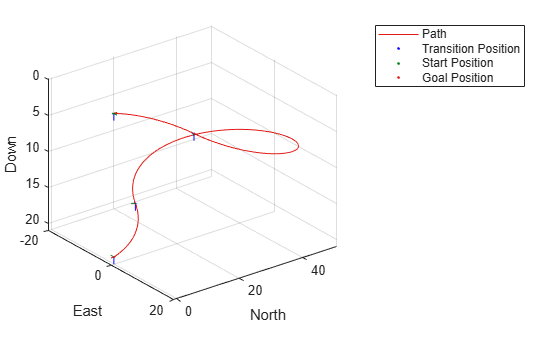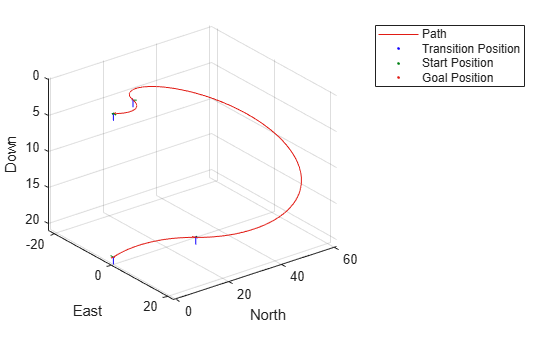# uavDubinsConnection

Dubins path connection for UAV

Since R2019b

## Description

The `uavDubinsConnection` object holds information for computing a `uavDubinsPathSegment` path segment to connect start and goal poses of a UAV.

A UAV Dubins path segment connects two poses as a sequence of motions in the north-east-down coordinate system.

The motion options are:

• Straight

• Left turn (counterclockwise)

• Right turn (clockwise)

• Helix left turn (counterclockwise)

• Helix right turn (clockwise)

• No motion

The turn direction is defined as viewed from the top of the UAV. Helical motions are used to ascend or descend.

Use this connection object to define parameters for a UAV motion model, including the minimum turning radius and options for path types. To generate a path segment between poses using this connection type, call the `connect` function.

## Creation

### Syntax

``connectionObj = uavDubinsConnection``
``connectionObj = uavDubinsConnection(Name,Value)``

### Description

example

````connectionObj = uavDubinsConnection` creates an object using default property values.```

example

````connectionObj = uavDubinsConnection(Name,Value)` specifies property values using name-value pairs. To set multiple properties, specify multiple name-value pairs.```

## Properties

expand all

Airspeed of the UAV, specified as a positive numeric scalar in m/s.

Data Types: `double`

Maximum roll angle to make the UAV turn left or right, specified as a positive numeric scalar in radians.

Note

The minimum and maximum values for `MaxRollAngle` are greater than `0` and less than `pi/2`, respectively.

Data Types: `double`

Flight path angle limits, specified as a two-element numeric vector ```[min max]``` in radians.

min is the minimum flight path angle the UAV takes to lose altitude, and max is the maximum flight path angle to gain altitude.

Note

The minimum and maximum values for `FlightPathAngleLimit` are greater than `-pi/2` and less than `pi/2`, respectively.

Data Types: `double`

UAV Dubins path types to disable, specified as a cell array of four-element character vectors or vector of string scalars. The cell array defines the four prohibited sequences of motions.

Motion TypeDescription
`"S"`

Straight

`"L"`

Left turn (counterclockwise)

`"R"`

Right turn (clockwise)

`"Hl"`

Helix left turn (counterclockwise)

`"Hr"`

Helix right turn (clockwise)

`"N"`

No motion

Note

The no motion segment `"N"` is used as a filler at the end when only three path segments are needed.

To see all available path types, see the AllPathTypes property.

Example: `{'RLRN'}`

Data Types: `string` | `cell`

Minimum turning radius of the UAV, specified as a positive numeric scalar in meters. This value corresponds to the radius of the circle at the maximum roll angle and a constant airspeed of the UAV.

Data Types: `double`

All possible path types, returned as a cell array of character vectors. This property lists all types. To disable certain types, specify types from this list in the DisabledPathTypes property.

For UAV Dubins connections, the available path types are: ```{'LSLN'} {'LSRN'} {'RSLN'} {'RSRN'} {'RLRN'} {'LRLN'} {'HlLSL'} {'HlLSR'} {'HrRSL'} {'HrRSR'} {'HrRLR'} {'HlLRL'} {'LSLHl'} {'LSRHr'} {'RSLHl'} {'RSRHr'} {'RLRHr'} {'LRLHl'} {'LRSL'} {'LRSR'} {'LRLR'} {'RLSR'} {'RLRL'} {'RLSL'} {'LSRL'} {'RSRL'} {'LSLR'} {'RSLR'}```.

Data Types: `cell`

## Object Functions

 `connect` Connect poses with UAV Dubins connection path

## Examples

collapse all

This example shows how to calculate a UAV Dubins path segment and connect poses using the `uavDubinsConnection` object.

Create a `uavDubinsConnection` object.

`connectionObj = uavDubinsConnection;`

Define start and goal poses as [`x`, `y`, `z`, `headingAngle`] vectors.

```startPose = [0 0 0 0]; % [meters, meters, meters, radians] goalPose = [0 0 20 pi];```

Calculate a valid path segment and connect the poses. Returns a path segment object with the lowest path cost.

`[pathSegObj,pathCosts] = connect(connectionObj,startPose,goalPose);`

Show the generated path.

`show(pathSegObj{1})`Display the motion type and the path cost of the generated path.

`fprintf('Motion Type: %s\nPath Cost: %f\n',strjoin(pathSegObj{1}.MotionTypes),pathCosts);`
```Motion Type: R L R N Path Cost: 138.373157 ```

This example shows how to modify an existing `uavDubinsPathSegment`object.

Connect Poses Using UAV Dubins Connection Path

Create a `uavDubinsConnection` object.

`connectionObj = uavDubinsConnection;`

Define start and goal poses as [`x`, `y`, `z`, `headingAngle`] vectors.

```startPose = [0 0 0 0]; % [meters, meters, meters, radians] goalPose = [0 0 20 pi];```

Calculate a valid path segment and connect the poses. Returns a path segment object with the lowest path cost.

`[pathSegObj,pathCosts] = connect(connectionObj,startPose,goalPose);`

Show the generated path.

`show(pathSegObj{1})`Verify the motion type and the path cost of the returned path segment.

`fprintf('Motion Type: %s\nPath Cost: %f\n',strjoin(pathSegObj{1}.MotionTypes),pathCosts);`
```Motion Type: R L R N Path Cost: 138.373157 ```

Modify Connection Type and Properties

Disable this specific motion sequence in a new connection object. Specify the `AirSpeed`, `MaxRollAngle`, and `FlightPathAngleLimit` properties of the connection object.

```connectionObj = uavDubinsConnection('DisabledPathTypes',{'RLRN'}); connectionObj.AirSpeed = 15; connectionObj.MaxRollAngle = 0.8; connectionObj.FlightPathAngleLimit = [-1.47 1.47];```

Connect the poses again to get a different path. Returns a path segment object with the next lowest path cost.

`[pathSegObj,pathCosts] = connect(connectionObj,startPose,goalPose);`

Show the modified path.

`show(pathSegObj{1})`Verify the motion type and the path cost of the modified path segment.

`fprintf('Motion Type: %s\nPath Cost: %f\n',strjoin(pathSegObj{1}.MotionTypes),pathCosts);`
```Motion Type: L R L N Path Cost: 164.674067 ```

 Owen, Mark, Randal W. Beard, and Timothy W. McLain. "Implementing Dubins Airplane Paths on Fixed-Wing UAVs." Handbook of Unmanned Aerial Vehicles, 2015, pp. 1677–1701.